# Innovative Teaching Methods In Formation Of Professional Competencies Of Future Mathematics Teachers

## Abstract

At this time, the Federal State Educational Standard of Higher Education, approved by the order of the Ministry of Education and Science of Russia, demand new requirements on results of education related to mastering the use of mathematical algorithms, including using modern computer systems. Therefore, the modern educational trend is aimed at introducing various types of information resources into the educational process of universities. The problem of this study is to find effective methods for using computer technology as a means of implementing the principle of visualization in the study of higher mathematics by future mathematics teachers. Software products are widely used for the teaching of various disciplines for professional and educational purposes. It is important to use mathematical software in universities to study mathematical disciplines. The study proposes a technique associated with the systematic use of mathematical software in the process of teaching higher mathematics. The article presents solutions to problems on the topic «Definite integral» using the graphic capabilities of mathematical software. The application of the proposed methodology during the sessions showed that the computer algebra system (CAS) Maple and GeoGebra are excellent tools for performing geometric constructions of the objects under study, the visualization of which facilitates solving the tasks for calculating the areas of plane figures and body volumes.

Keywords: Definite integralmathematical softwareprinciple of visualization

## Introduction

According to the objectives of the State Program of the Russian Federation "Development of Education" for the period of 2013-2020, the amount of teachers using modern information and communications technologies should increase (The State Program of the Russian Federation, 2014). At this time, the Federal State Educational Standard of Higher Education, approved by the order of the Ministry of Education and Science of Russia, demand new requirements on results of education related to mastering the use of mathematical algorithms, including using modern computer systems. Therefore, the modern educational trend is aimed at introducing various types of information resources into the educational process of universities.

Higher mathematics is studied at each university and it is as a basis for higher education. This subject is compulsory for the students to learn regardless of the direction of the bachelor's programs.

It is mathematical disciplines that are one of those subjects which the principle of visualization is effectively realized. This allows to form and develop figurative, abstract, visual, spatial thinking of students, which forms the professional competencies of future mathematics teachers.

Software products are widely used for the teaching of various disciplines for professional and educational purposes [Gainutdinova & Shirokova, 2016]. It is significant to use mathematical software in universities in the study of mathematical disciplines. At the same time, the teacher builds on the previously studied school material and uses it in a new format to systematize the knowledge of students. The reason of it is that university knowledge should be more profound and meaningful.

The article describes the opportunities for realizing the principle of visualization in the studying of the topic “Definite integral” using ICT. The principle of visualization is the leading didactic principle of teaching. Its necessity is based on the dialectic of the move from visual perception to abstract thinking in the process of understanding. At the heart of the principle of visualization is the imagining of abstractions.

## Problem Statement

The problem of this study is to find effective methods for using computer technology as a means of implementing the principle of visualization in the study of higher mathematics by future mathematics teachers.

The effectiveness of the perception of new material depends on essential ways of educational processes. That is why it is necessary to pay serious attention to the methodology of teaching mathematical disciplines using information technology.

The introduction of information and communication tools is difficult from the point of view of the methodological and pedagogical aspects of teaching mathematics in universities. In addition, the complexity of the methodological problem of teaching is related to the fact that the content of the disciplines has been subjected to great changes in the direction of reducing the number of hours given to them.

Great opportunities for better study of the material are opened by the use of mathematical software’s (Denisova, 2016). These include Maple, Mathematic, GeoGebra (Dyakonov, 2006; 2008; 2011; Larin, 2015), and others. It is known that all of them possess powerful graphic capabilities of spatial modelling of objects, including animating graphs - animation. In addition, they easily cope with routine tasks such as; solving algebraic and differential equations, analysing functions, numerical and analytical differentiation and integration.

Along with the abovementioned, the paper considers the solution of a number of problems for calculating the areas of plane figures and solid volumes using the computer mathematics systems Maple and GeoGebra.

## Research Questions

There are frequent problems with considering applications of definite integral, for example, when calculating areas of plane figures and volumes of a solid at the stage of constructing the objects. Students cannot always plot the sought-for region or cannot deﬁne its boundaries during the process of evaluation areas of flat figures. Sometimes students can’t even start solving the problem, not to talk of calculating the integral because they cannot imagine the solid while calculating volumes. Therefore, the research questions consist of applying the methodology based on the geometric constructions of the objects under study, the imagining of which makes it possible to easily solve the problems posed. This methodology enables the solution of complex mathematical problems, developing research capabilities, thereby putting the elements of experiment and scientific research into the educational process.

## Purpose of the Study

The aim of the research is developing the skills of students in solution of problems in higher mathematics using information and communication technologies (ICT).

## Research Methods

To consider the solution to a number of problems for calculating the areas of plane figures and solid volumes using the computer mathematics systems Maple and GeoGebra

## Methods for visualizing the solution of problems in Geogebra software.

Methods for visualizing the solution of problems in Geogebra software is used in the examples 1-3 below [Berman, 2005].

Example 1. Calculate the area of a curvilinear trapezoid bounded by a line

$\mathrm{f}\left(\mathrm{x}\right)={\mathrm{e}}^{-\mathrm{x}}\left({\mathrm{x}}^{\mathrm{2}}+\mathrm{3}\mathrm{x}+\mathrm{1}\right)+{\mathrm{e}}^{\mathrm{2}}$, Ox axis and two lines parallel to the axes Oy, drawn through the points of the extremum of the function y.

The solution. To determine the boundaries of integration, it is necessary to find the extremum points of a given function. This can be done manually, by examining the function, which will take a lot of time. The capabilities of the GeoGebra software are used to solve the task. The required extremum points will be found by applying the tool.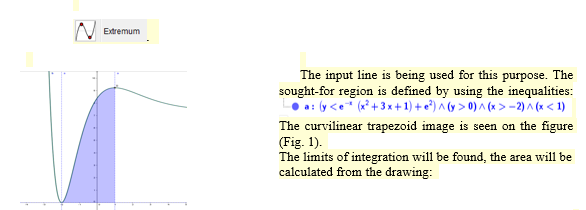Figure 1: [Curvilinear trapezoid in GeoGebra]

$S = ∫ - 2 1 e - х ( x 2 + 3 x + 1 + e 2 ) d x = 3 e 2 - 12 e - 1$

Example 2. Calculate the areas, bounded by lines y $=\mathrm{l}\mathrm{n}\mathrm{x}$ and $\mathrm{y}={\mathrm{l}\mathrm{n}}^{\mathrm{2}}\mathrm{x}$.

The solution. The sought-for region bounded by given curves is constructed in the GeoGebra packet.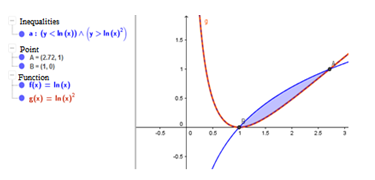Figure 2: [Image of a flat figure in GeoGebra]

The borders of integration will be found from the figure

$S = ∫ 0 1 e y - e y d y = = 3 - e$

Example 3. Find the volume of the body bounded by the conic surface ${\left(\mathrm{z}\mathrm{}-\mathrm{2}\right)}^{\mathrm{2}}=\mathrm{}\frac{{\mathrm{x}}^{\mathrm{2}}}{\mathrm{3}}\mathrm{}+\mathrm{}\frac{{\mathrm{y}}^{\mathrm{2}}}{\mathrm{2}}\mathrm{}\mathrm{}$ and the plane $\mathrm{z}=\mathrm{0}$.

The solution. The GeoGebra software is used. The equations of the surfaces will be given and will be founded the section of the cone by the plane $\mathrm{}\mathrm{z}=\mathrm{0}\mathrm{}$for the construction of a solid in the input line. The following will be gotten: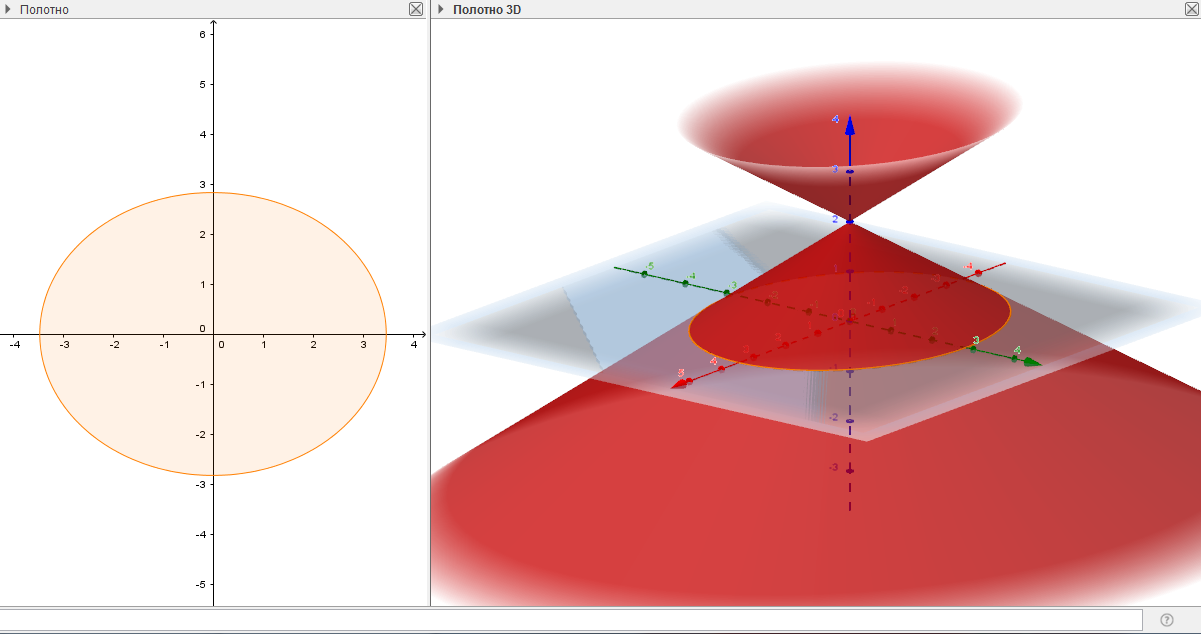Figure 3: [Section of a cone by a plane]

The limits of integration are found from the figure.

$V = 4 ∫ 0 12 d x ∫ 0 4 3 ( 12 - x 2 ) 2 - x 2 3 + y 2 2 d y = 8 6 π 3$

## Methods for visualizing the solution of problems in Maple software.

The Maple software is used in the examples 4-7 [Romanova & Trachevskaya, 2005].

Example 4. Calculate the areas, bounded by the lines $\mathrm{}\mathrm{у}=\mathrm{1}-{\mathrm{x}}^{\mathrm{2}}$ and $\mathrm{у}={\left(\mathrm{x}-\mathrm{1}\right)}^{\mathrm{2}}$.

The solution. The figure of the calculating areas is plotted: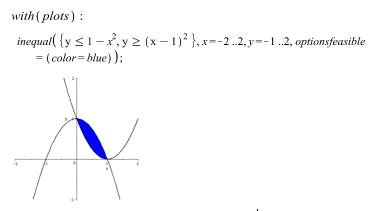Figure 4: [Image of a flat figure]

The command solve must be applied to find the intersection points of the curves:

$\text{solve}\left(\left\{\mathrm{y}=\mathrm{1}-{x}^{\mathrm{2}},\mathrm{y}=\left(\mathrm{x}-\mathrm{1}{\right)}^{\mathrm{2}}\right\},\left\{x,y\right\}\right)$

${ x = 0 , y = 1 } , { x = 1 , y = 0 }$

The area of the required figure is founded by the determination the limits of integration:

$S = ∫ 0 1 1 - x 2 - x - 1 2 d x = 1 3$

Example 5. Calculate the areas, bounded by the lines $\mathrm{у}=-\mathrm{x}-\mathrm{2}$, $\mathrm{у}=\mathrm{x}-\mathrm{2}$ and $\mathrm{у}=\mathrm{2}$.

The solution. The command intersection must be applied to construct the given lines and find their intersection points

[The image of the desired shape]

The areas of the sought-for figure will be calculated with the determining the limits of integration:

$V = ∫ - 2 2 y + 2 - - y - 2 d y = 16$

Example 6. Calculate the volume of a solid bounded by an elliptic paraboloid $\frac{{\mathrm{x}}^{\mathrm{2}}}{\mathrm{4}}+\frac{{\mathrm{y}}^{\mathrm{2}}}{\mathrm{2}}=\mathrm{z}$ and the plane $\mathrm{z}=\mathrm{1}$.

The solution. It will be determined what the intersection of these surfaces is.

The image of the elliptic paraboloid and the plane will be obtained using the plot3d function.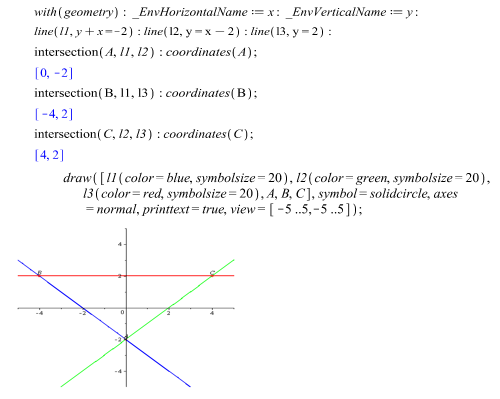Figure 5: [Solid image from different angles]

It should be noted that the line of intersection of these surfaces is an ellipse. It is necessary to find the equation of the ellipse, solving jointly the equations of the paraboloid and the plane:

$\frac{{\mathrm{x}}^{\mathrm{2}}}{\mathrm{4}}+\frac{{\mathrm{y}}^{\mathrm{2}}}{\mathrm{2}}=\mathrm{1}$.

The implicitplot function should be used to construct a two-dimensional graph.

The contour of the projection of the intersection of surfaces on the Oxy axis is an ellipse with semiaxes equal to 2 and $\sqrt{\mathrm{2}}$.

The volume will be found using the integral results of the construction.

The accuracy of the results should be checked using Maple.

$2 π$

Example 7. Calculate volume of solid, bounded by these surfaces, using triple integral $\mathrm{z}=\mathrm{10}-{\mathrm{x}}^{\mathrm{2}},\mathrm{}\mathrm{}\mathrm{z}=\mathrm{0},\mathrm{}{\mathrm{x}}^{\mathrm{2}}{+\mathrm{y}}^{\mathrm{2}}=\mathrm{4}$

The solution. Plot these surfaces (Fig. 8).

restart $:$ with $\left($ plottools $\right):$ with $\left($ plots $\right):$ with $\left($ student $\right):$ $Q I : = c y l i n d e r ⁡ ( [ 0,0 , 0 ] , 2 , . 01 ) :$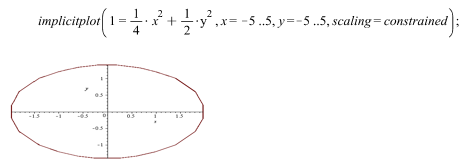Figure 7: [Visualization of a given solid]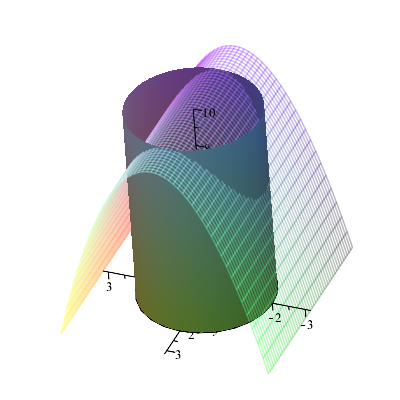Figure 8: [Visualization of a quarter solid]

It is possible to confine to the image of a quarter of the body. From the symmetry of the figure relative to the Ox and Oy axes, the volume of which is required to be found (Fig. 9):

restart $:$ with $\left($ plottools $\right):$ with $\left($ plots $\right):$ with $\left($ student $\right):$

$QI:=$ cylinderplot $\left(\mathrm{2},\theta =\mathrm{0}..\pi /\mathrm{2},z=\mathrm{0}..\mathrm{0.1}\right):$ plots $\left[$ display $\right]\left(\left[Q\mathrm{1},Q\mathrm{2},Q\mathrm{3},Q\mathrm{4},Q\mathrm{5}\right]$, axes $=$ normal, orientation $=\left[\mathrm{116},$, $63 ] ) ;$

It is necessary to go into a cylindrical coordinate system and calculate the volume:

restart : with ( plottools): with ( plots):with (student) : $a s i g ⁡ n ( x = ρ ⋅ c o s ⁡ ( φ ) , y = ρ ⋅ s i n ⁡ ( φ ) , z = z I ) ;$ $z : = 10 - x 2$ $z : = - ρ 2 c o s ⁡ ( φ ) 2 + 10$ $\mathrm{V}=\mathrm{4}\cdot$ Tripleint $V = 4 ∫ 0 1 2 π ∫ 0 2 ∫ 0 - p 2 c o s ⁡ ( φ ) 2 + 10 ρ d z 1 d ρ d φ$ $V=$ value $\left(\mathrm{4}\cdot$ Tripleint $V = 36 π$

## Findings

The introduction of modern information technologies in the educational process allows for the creation of computer tools to enhance learning processes. They are effectively used for modelling and creating mathematical concepts. The results of the study showed that using the methods help to improve the quality and effectiveness of the studied material.

There was a method which allowed us to test the effectiveness of modern information technologies in the classes on higher mathematics. This technique is closely related with the systematic usage of mathematical packages in the process of teaching higher mathematics to students. There are conclusions which were drawn as a result of the research, that mathematical software:

• increases the level of mastering the material of complicated sections of higher mathematics;

• allows for conducting geometrical plot of the objects under study, visualization of which facilitates the solution of the problem;

• has powerful graphical capabilities allowing not only to visualize the objects, but also to see them in dynamics;

• a means of developing of spatial thinking, effectively using the principle of visibility;

• enables the solution of complex mathematical problems, developing research capabilities, thereby making the elements of experiment and scientific research into the educational process;

• effective tools for achieving the inter-subject connections of mathematics with other subjects;

• allows optimizing the learning process, using time rationally at different stages of learning;

• allows organizing the educational process, introducing a differentiated approach to teaching;

increases the interest of students in learning activity and interest in its final result.

## Conclusion

The use of information and communication technologies has a great influence on the content, forms and methods of teaching. The principles of visualization and computer technologies are closely interrelated and their combination competent leads to an improvement in the quality of knowledge of students.

The study proposes a technique associated with the systematic use of mathematical software in the process of teaching higher mathematics. The article presents solutions to problems on the topic «Definite integral» using the graphic capabilities of mathematical software. The application of the proposed methodology during the sessions showed that the computer algebra system (CAS) Maple and GeoGebra are an excellent tool for performing geometric constructions of the objects under study, the visualization of which facilitates solving the tasks for calculating the areas of plane figures and body volumes.

Teacher makes it possible to individualize the learning process using a CAS to teach the course of higher mathematics. The use of computer tools in the teaching of mathematical disciplines makes it possible to effectively use new information technologies in the educational process and translate teaching to a higher level.

## Acknowledgments

The work is performed according to the Russian Government Program of Competitive Growth of Kazan Federal University.

## References

1. Berman, G. N. (2005). Collection of problems on the course of mathematical analysis. Moscow: Science.
2. Denisova, M. (2016). Application of the GeoGebra interactive environment in the study of a definite integral. VI International Scientific-Practical Conference “Mathematics education in school and university: theory and practice”, 218-220.
3. Dyakonov, V. (2011). Maple 10/11/12/13/14 in mathematical calculations.
4. Dyakonov, V. (2006). Maple 9.5 / 10 in mathematics, physics and education.
5. Dyakonov, V. (2008). Mathematic 5.1/5.2/ 6 in mathematical and scientific-technical calculations.
6. Gainutdinova, T. & Shirokova, O. (2016). Features of Professional Teachers Training of Informatics in a Programming Course. International Forum on Teacher Education Collection of IFTE 2016 Volume XII, The European Proceedings of Social & Behavioral Sciences EpSBS, 30-37.
7. Larin, S. (2015). Computer animation in the environment of GeoGebra in the lessons of mathematics: a textbook.
8. Romanova, O. & Trachevskaya Z. (2005). Multiple integrals in the Maple system. Series: Information systems and logic, (3).
9. The State Program of the Russian Federation "Development of Education" for 2013-2020 (2014). Government of the Russian Federation.

31 August 2017

#### Article Doi

https://doi.org/10.15405/epsbs.2017.08.02.24

#### eBook ISBN

978-1-80296-028-0

29

-

1st Edition

1-960

#### Subjects

Teacher, teacher training, teaching skills, teaching techniques